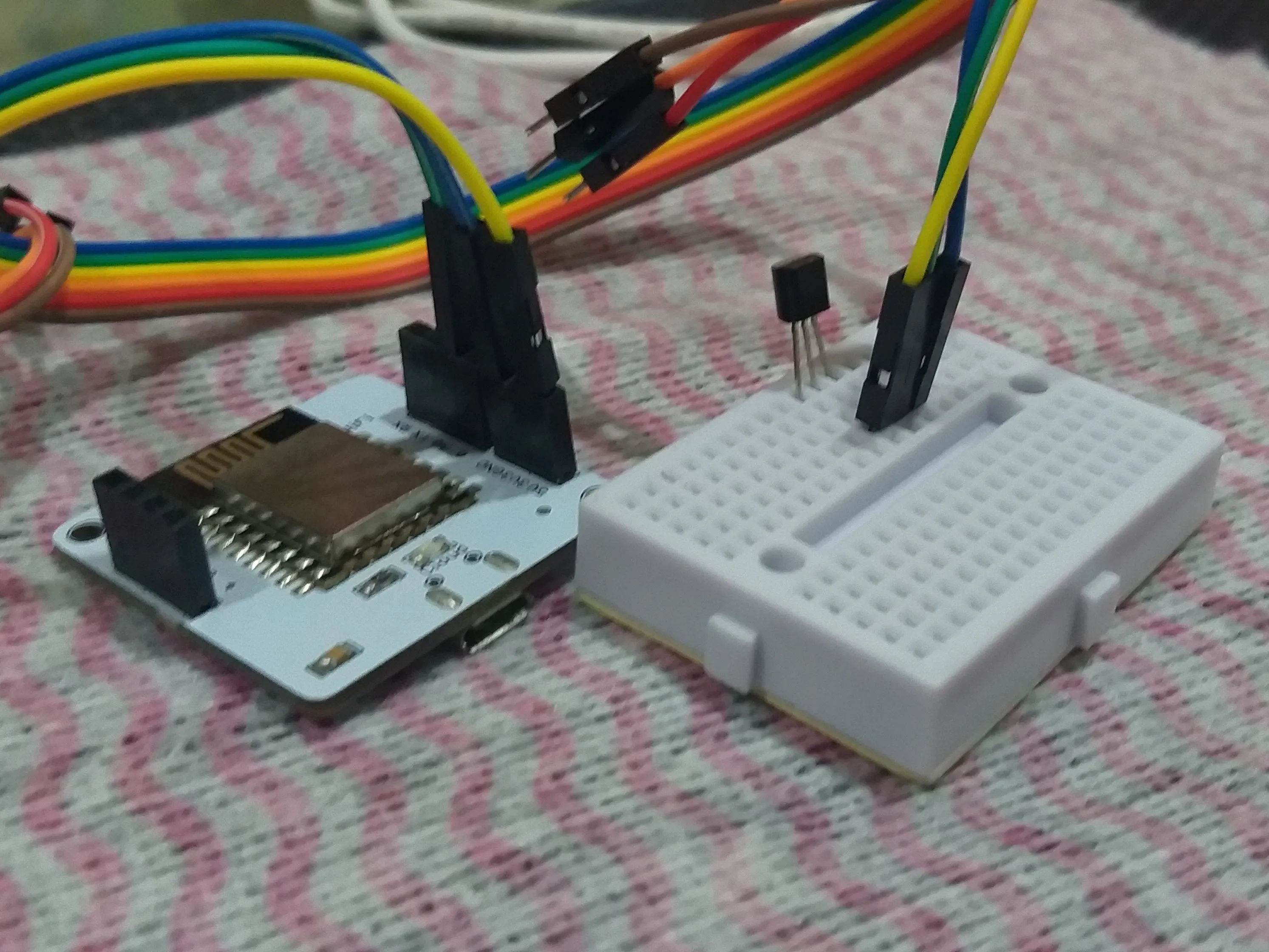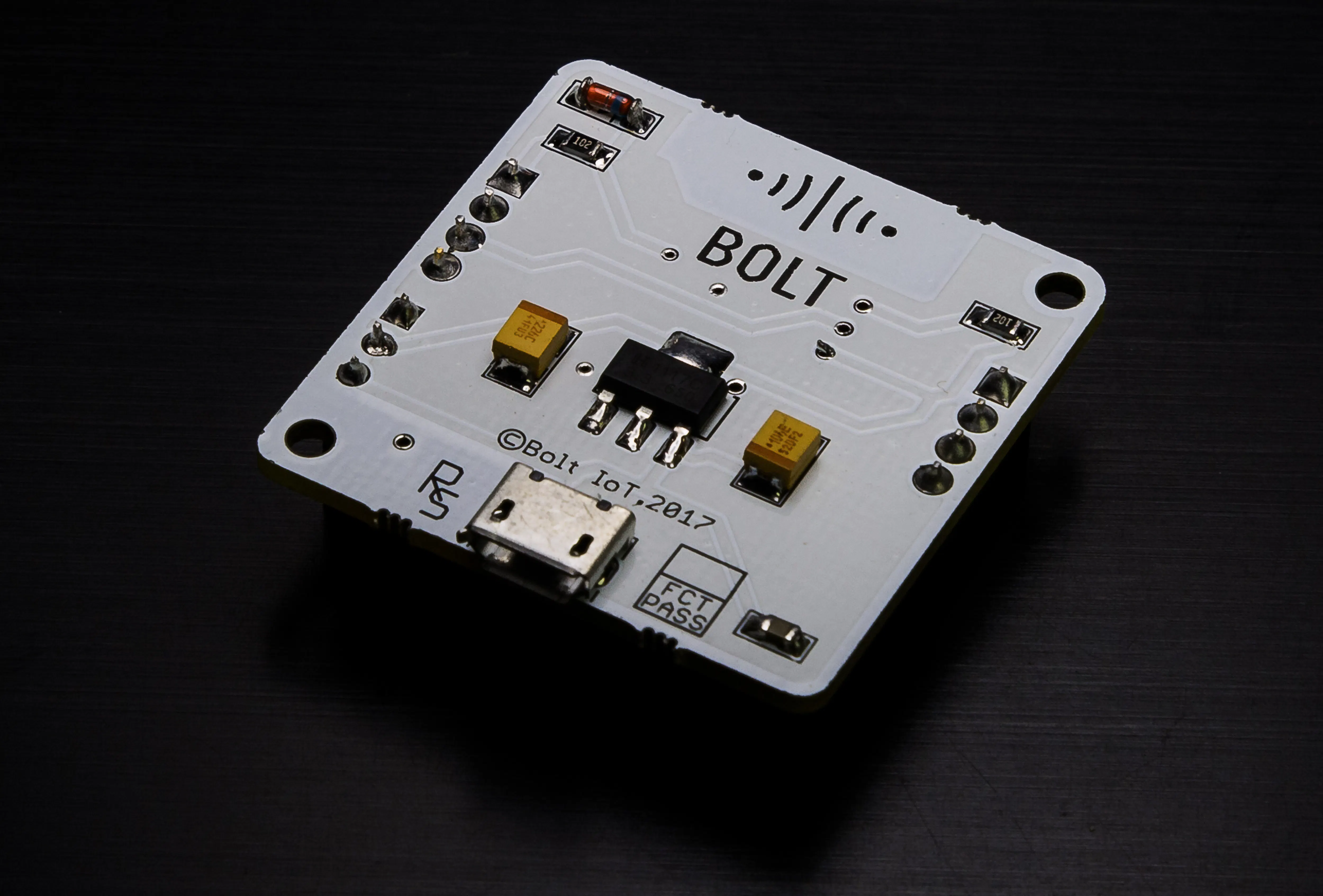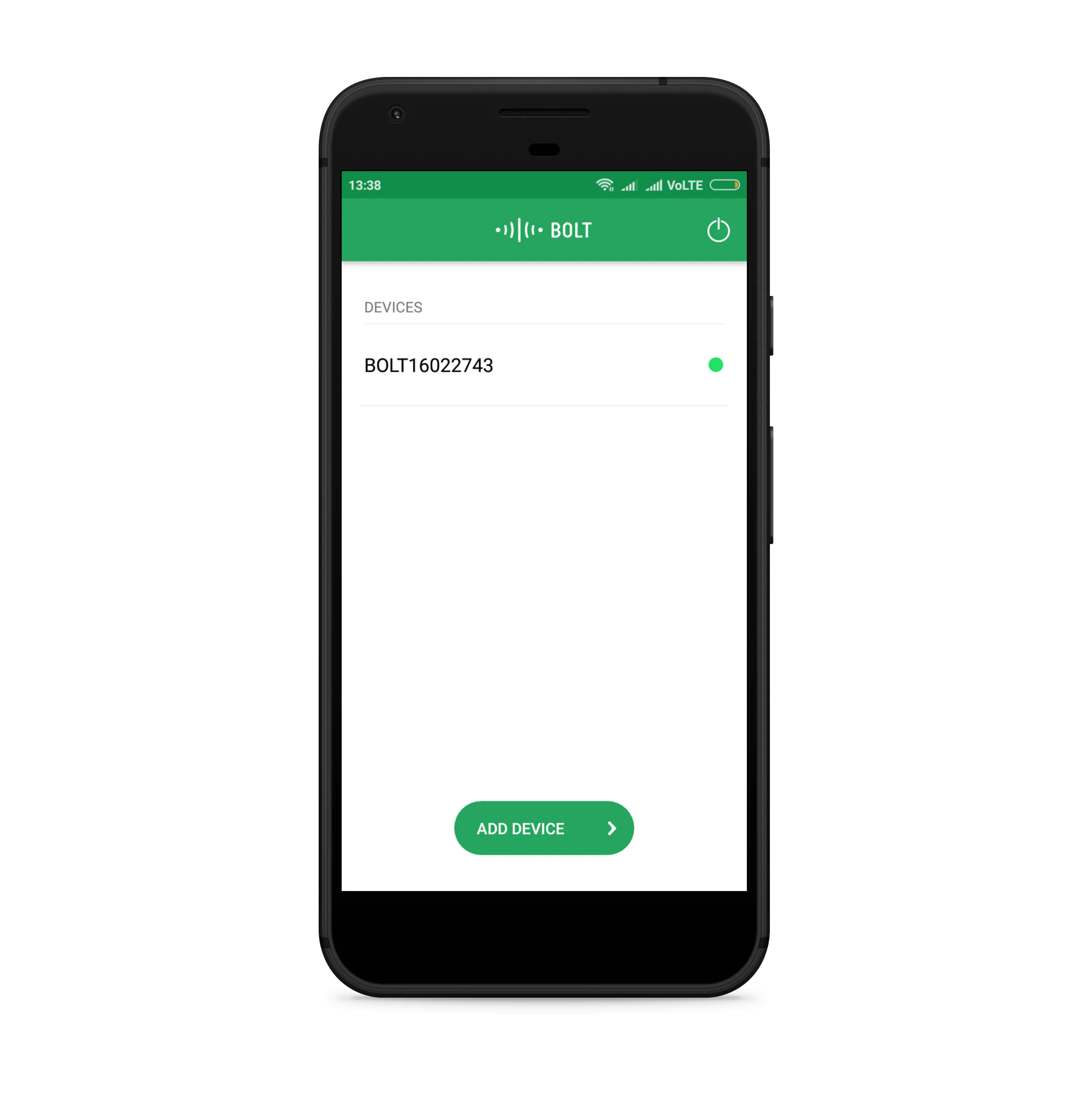Published

# Indoor Temperature Monitoring System

Internet of Things based temperature monitor with predictive capabilities & anomaly detection using ML algorithms.## Things used in this project

### Hardware componentsBolt IoT Bolt WiFi Module
×1
 LM35 Temperature Sensor
×1Jumper wires (generic)
×1×1Micro-USB to USB Cable (Generic)
×1
 Portable Power Bank
×1

### Software apps and online servicesBolt IoT Bolt CloudBolt IoT Android App
 MailgunSnappy Ubuntu Core

## Code

### Temperature-monitor

Python
```import conf, json, time, math, statistics
from boltiot import Email, Bolt

max_lim = 299
min_lim = 10

def compute_bounds(history_data,frame_size,factor):
if len(history_data)<int(frame_size) :
return None

if len(history_data)>int(frame_size) :
del history_data[0:len(history_data)-int(frame_size)]
Mn=statistics.mean(history_data)
Variance=0
for data in history_data :
Variance += math.pow((data-Mn),2)
Zn = int(factor) * math.sqrt(int(Variance) / int(frame_size))
High_bound = history_data[int(frame_size)-1]+Zn
Low_bound = history_data[int(frame_size)-1]-Zn
return [High_bound,Low_bound]

tempo = Bolt(conf.API, conf.DEVICE_ID)
mailer = Email(conf.MAILGUN, conf.SANDBOX, conf.SENDER_EMAIL, conf.RECIPIENT_EMAIL)
history_data=[]

while True:
print("Collecting sensor value")
print("Sensor value " +str(data['value']))
try:
sensor_value = int(data['value'])
if sensor_value>max_lim or sensor_value<min_lim:
print("Making request to Mailgun to send an email")
respo = mailer.send_email("Alert: Temprature exceeded thesold limit.")
print("Response received from Mailgun is: " +str(response_text['message']))
except Exception as e:
print ("Error occured: Below are the details")
print (e)
time.sleep(10)

bound = compute_bounds(history_data,conf.FRAME_SIZE,conf.MUL_FACTOR)
if not bound:
required_data_count=int(conf.FRAME_SIZE)-len(history_data)
print("Not enough data to compute Z-score. Need ",required_data_count," more data points")
history_data.append(int(data['value']))
time.sleep(10)
continue
try:
if sensor_value>bound :
print ("Someone has opened the door. Sending an Email")
response1 = mailer.send_email("Alert","Someone opened fridge door")
print("This is the response ",response1)
history_data.append(sensor_value);
except Exception as e:
print ("Error",e)
time.sleep(10)
```

### Prediction

JavaScript
```setChartLibrary('google-chart');
setChartTitle('Polynomial Regression');
setChartType('predictionGraph');
setAxisName('time_stamp','temp');
mul(0.0977);
plotChart('time_stamp','temp');
```

## Credits

### Divnoor Singh

1 project • 0 followers